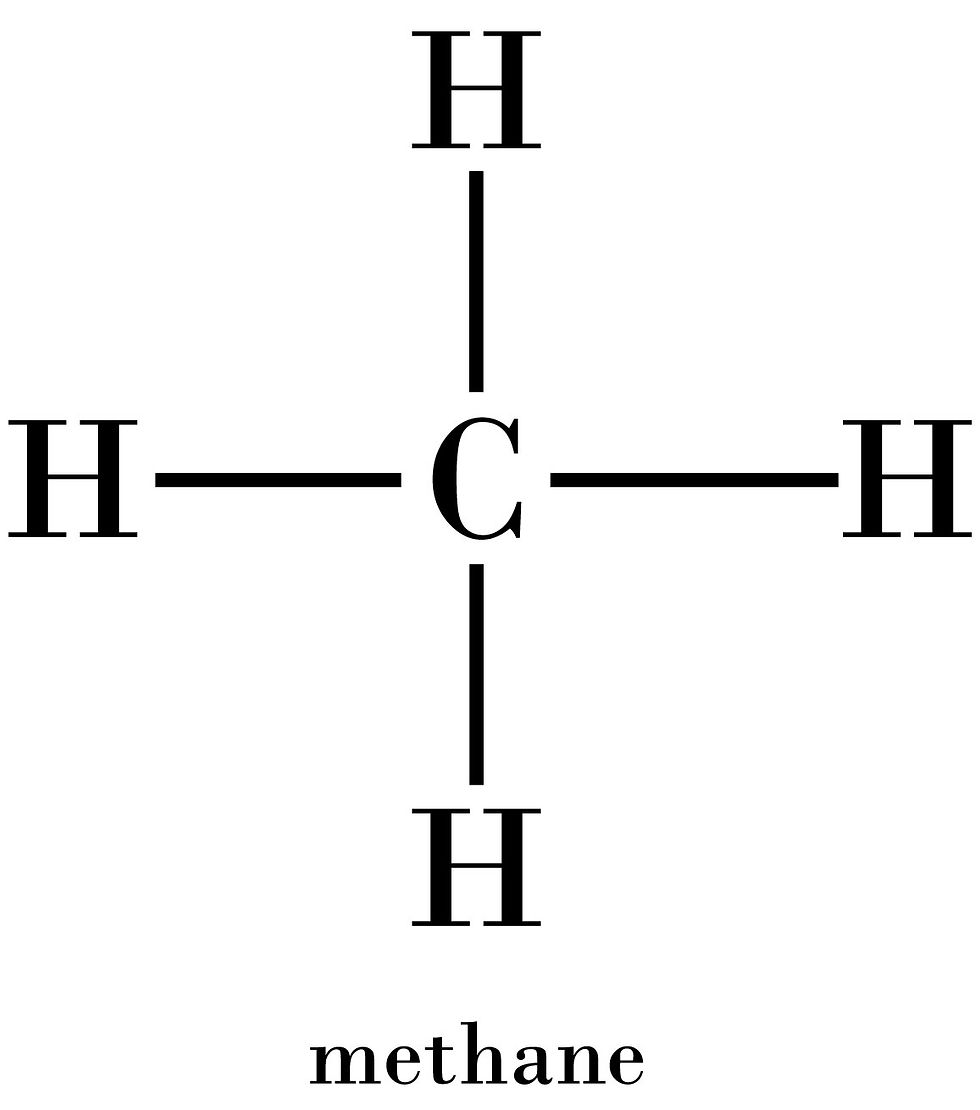cum
top of page
Search

# CSEC Chemistry: Types of Organic Formulae and Homologous Series

Updated: Jul 25, 2020

Formulae of Organic Compounds

Organic compounds can be represented through five different types of formulae:

• molecular formula

• empirical formula

• full structural (displayed) formula

• condensed structural formula

• general formula

(Molecular and empirical formulae will not be discussed in this post, as they do not give any information regarding the arrangement of atoms relative to each other.)

Structural/Displayed Formula

The full structural or displayed formula of an organic compound represents how all the atoms in the molecule are bonded to one another. All bonds are shown in two dimensions, and thus do not indicate the actual shape of the molecule or the positions of the atoms.

For example:Condensed Structural Formula

This gives a bit less information than the displayed formula. It shows the atoms relative to one another, but does not show the individual bonds. Whenever looking at condensed formulae, just remember that each carbon atom always forms 4 total bonds in organic compounds.General Formula

The general formula represents a group of similar organic compounds called a homologous series. It gives the numerical relationship between the number of carbon atoms and the other types of atoms in one molecule of any of the compounds in a given homologous series.

For example, the general formula of compounds of the homologous series alkanes is CnH2n+2

Homologous Series

Homologous series were mentioned above, but you may not be familiar with them. These are groups of organic compounds which share similar characteristics (i.e. physical properties and chemical structures) which differ only by the number of carbon atoms in the structural backbone. The members of a homologous series are arranged in ascending order of molecular mass.

Characteristics of a homologous series:

• Each member has the same general formula.

• All members of the series have similar chemical properties.

• The physical properties of members of a homologous series change in a regular way as the length of the carbon chain in the compound increases.

• Each member's molecular mass differs from the member before and after it by one CH2 unit (molecular mass= 14).

• All members of a homologous series may be prepared by the same general method.

The general formulae of the homologous series:In each formula, the number of carbon atoms is represented by n, which is a whole number value. In carboxylic acids, esters and aldehydes, n may also have a value of zero.

So, now that we know the general formulae of the homologous series, we should be able to deduce the homologous series of a certain compound when given its molecular formula. For example, given that the formula for methane is CH4, n would be 1 and 4 = 2(1) +2. Thus, methane is an alkane.